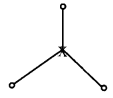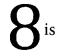# MCQ Questions for Class 7 Maths Chapter 14 Symmetry with Answers

We have compiled the NCERT MCQ Questions for Class 7 Maths Chapter 14 Symmetry with Answers Pdf free download covering the entire syllabus. Practice MCQ Questions for Class 7 Maths with Answers on a daily basis and score well in exams. Refer to the Symmetry Class 7 MCQs Questions with Answers here along with a detailed explanation.

## Symmetry Class 7 MCQs Questions with Answers

Choose the correct option.

Question 1.
How many lines of symmetry are there in a regular pentagon?
(a) 1
(b) 2
(c) 3
(d) 5

Question 2.
How many lines of symmetry are there in a rectangle?
(a) 1
(b) 2
(c) 3
(d) 4

Question 3.
The order of rotational symmetry of a circle is
(a) 1
(b) 4
(c) 2
(d) infinite

Question 4.
The order of rotational symmetry of the following figure is:(a) 1
(b) 2
(c) 3
(d) 4

Question 5.
Which of the following has only one line of symmetry?
(a) A rectangle
(b) A square
(c) A semi circle
(d) An equilateral triangle

Question 6.
The angle of rotation of an isosceles triangle is
(a) 120°
(b) 270°
(c) 180°
(d) 360°

Question 7.
How many lines of symmetry are there in the following figure?(a) 1
(b) 2
(c) 3
(d) 4

Question 8.
How many lines of symmetry are there in a scalene triangle?
(a) 1
(b) 0
(c) 2
(d) 4

Question 9.
How many lines of symmetry are there in a parallelogram?
(a) 0
(b) 1
(c) 2
(d) None of these

Question 10.
The order of the rotational symmetry of the following figure about the point marked x is(a) 1
(b) 2
(c) 3
(d) 4

Question 11.
Which of the following has rotational symmetry?Question 12.
The order of rotational symmetry of a circle is:
(a) 1
(b) 4
(c) 2
(d) infinite

Fill in the blanks

Question 1.
The rotational symmetry of orderQuestion 2.
A figure which can be rotated into itself is said to have ………………….. symmetry.

Question 3.
The point about which the figure is rotated is called the ………………….. of rotational symmetry.

Question 4.
The number of times a figure fits into itself in one complete rotation is called the order of ………………….. symmetry.

Question 5.
A rectangle has rotational symmetry of order …………………..

Question 6.
A figure is said to be symmetrical about a …………………. if it is identical on either side of it. [plane/line]

Question 7.
An isosceles triangle is symmetrical about the bisector of the angle included between the …………………. sides. [equal/unequal]

Question 8.
If ABCD is an isosceles trapezium such that AB || DC and AD = BC, then it is symmetrical about the line joining the mid-point of …………………. [AB and DC/AD and BC]

Question 9.
A rectangle has two lines of symmetry each one of which is the line joining the mid-point of …………………. sides. [opposite/adjacent]

Question 10.
The number of times a shape will fit onto itself in one complete turn is called …………………. of the rotational symmetry.

Question 11.
An equilateral triangle has …………………. symmetry of order three.

Question 12.
Rotating a figure through 90° clockwise is the same as rotating it anti-clock wise through …………………. [270°/180°]

Question 13.
Rotating a figure through 180° clockwise is the same as rotating it anti-clock wise through …………………. [90°/180°]